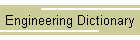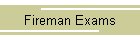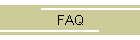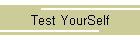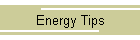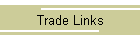Work exists only in transitional form. Work is equal to the product of the force used to move something and the distance that it is moved. If there is no movement there is no work done. ```W = F D W Work F Force D Distance```In a steam engine the pressure of the steam acting upon a piston does work. The force is the product of that pressure and the area of the piston. ```F = p A p pressure A Area pressure is acting upon```The work done by the piston is equal to the force times the distance the piston moves. ```W = p A d d Distance the piston moves (Stroke)```Since A d is the displacement volume v of the piston. Work can be expressed in terms of the displacement. ```W = p v v Volume displaced by piston.``` ``` ```